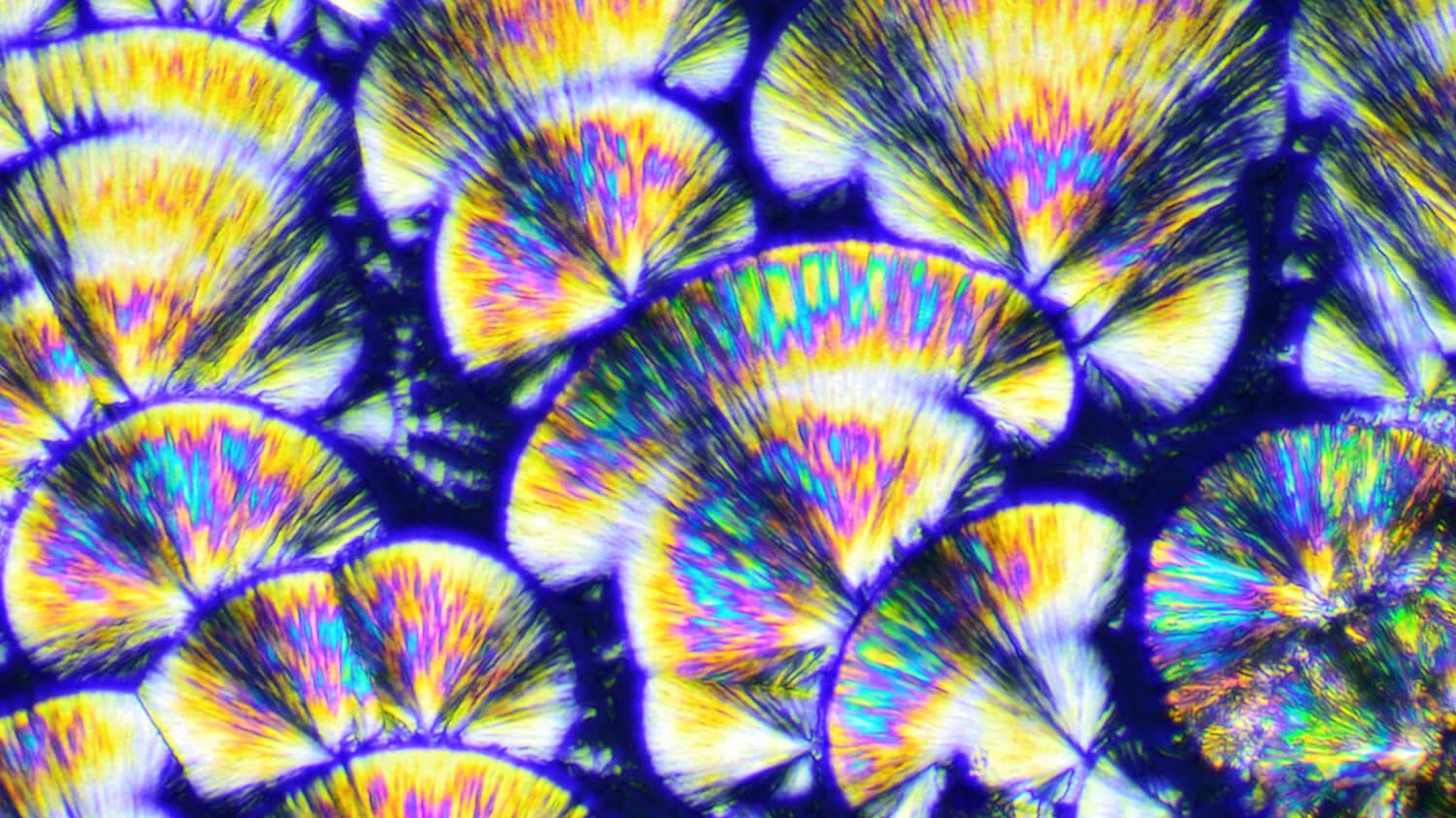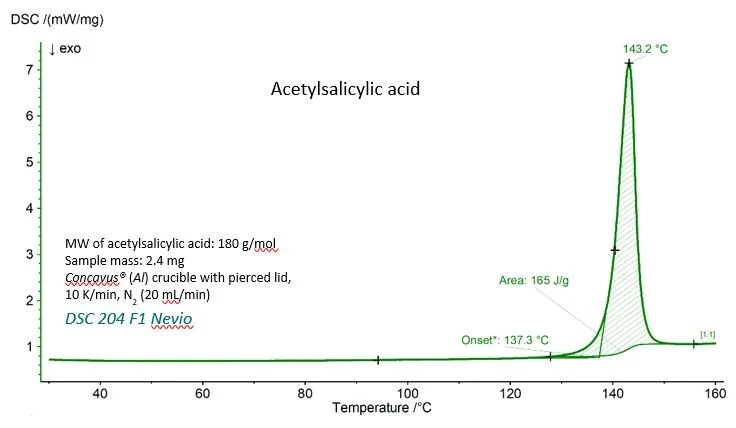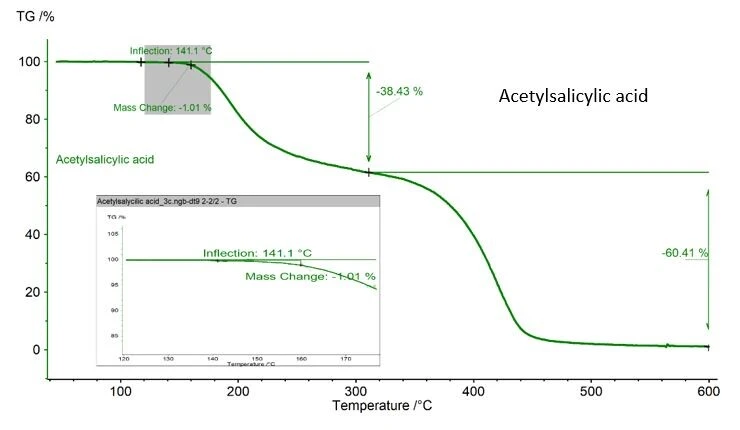27.03.2023 by Dr. Ligia de Souza

# DSC for Determining the Ideal Solubility? Tell Me How!

Differential scanning calorimetry (DSC) is a widely used analytical technique in the pharmaceutical industry for investigating the thermal properties of drug substances. One of the key applications of DSC is to determine the ideal solubility of a drug, which is crucial for developing effective and safe pharmaceutical formulations. In this article, we will explore how DSC can be used to determine the ideal solubility of drugs, and the factors that can influence the solubility behavior. Whether you are a researcher, scientist, or formulator in the pharmaceutical industry, this article will provide you with valuable insights on the use of the DSC instrument by NETZSCH Analyzing & Testing for determining ideal solubility. So, let's dive into this topic!

Classification of Drugs Based on Solubility

Aqueous solubility is essential for a drug to reach its therapeutic target, given that the dissolution rate directly influences the drug bioavailability. The United States Pharmacopoeia and European Pharmacopoeia classify drugs based on their approximate solubility range in mg/ml. For example, 100–1000 mg/ml is the solubility range for a molecule considered freely soluble, and 0.1–1 mg/ml is the range for a drug molecule characterized by very slight water solubility. Therefore, determining aqueous and non-aqueous solubility will define the best possible formulation approach for a good drug candidate.

Ideal solubility gives the saturated concentration of a solute, in mole fraction, when an ideal solvent is used, i.e., the theoretical case of a solute being dissolved in a solvent without any loss of energy during the dissolution process. In practice, this is not achievable because the solute-solvent interaction is usually non-ideal and the chemical interaction between the solute and the solvent may hinder the dissolution process. Examples of these intermolecular interactions are hydrogen bonds, dielectric properties, and dipole moment.

While the method of choice for determining the solubility of a molecule is UV spectrophotometry, the ideal solubility can be calculated if the Melting Temperatures and EnthalpiesThe enthalpy of fusion of a substance, also known as latent heat, is a measure of the energy input, typically heat, which is necessary to convert a substance from solid to liquid state. The melting point of a substance is the temperature at which it changes state from solid (crystalline) to liquid (isotropic melt).melting point and enthalpy of fusion of the substance are known.

However, What Does Ideal Solubility Mean in Thermodynamic Terms?

In the dissolution process, the solute-solute bonds must be broken. The energy input required to break these bonds is equal to the energy needed to melt a solid; i.e., the enthalpy of fusion, (∆Hf). On the other hand, solvent-solvent bonds must also be broken while solute-solvent bonds must be formed. The energy input for this last step can be called enthalpy of mixing (∆Hmix). Thus, the enthalpy of dissolution is the sum of the enthalpy of fusion and the enthalpy of mixing:

∆Hsol = ∆Hf + ∆Hmix

If the enthalpy of mixing equals zero, then the enthalpy of dissolution equals the enthalpy of fusion:

∆Hsol = ∆Hf

These are the main thermodynamic assumptions for the ideal dissolution of a crystalline material. Ideal dissolution leads to ideal solubility.

Other assumptions are that ∆Hf is positive (fusion is an EndothermicA sample transition or a reaction is endothermic if heat is needed for the conversion.endothermic event) and so is ∆Hsol. However, in order for a spontaneous reaction to occur, the Gibbs free energy (∆G = ∆Hf  -T∆S)must be negative; thus, the entropy (S) must be positive. Considering that the Melting Temperatures and EnthalpiesThe enthalpy of fusion of a substance, also known as latent heat, is a measure of the energy input, typically heat, which is necessary to convert a substance from solid to liquid state. The melting point of a substance is the temperature at which it changes state from solid (crystalline) to liquid (isotropic melt).melting temperature and enthalpy of fusion are independent of the experimental temperature, and that the dissolution will provide a saturated solution, the Van’t Hoff equation can be applied as follows:

Where:  x2 = saturated concentration of the drug in mole fraction units

∆Hf = enthalpy of fusion (J/mol)

R = gas constant (J/K∙mol)

T = given temperature (K)

The result yields the saturated concentration of a solute in the ideal solvent, in mole fraction. In other words, this would be the maximum achievable concentration of the drug in the best possible solvent. Aulton’s Pharmaceutics book  cites the acetylsalicylic acid example. The (calculated) ideal solubility of acetylsalicylic acid is 0.037 mole fraction; the best solvent listed is tetrahydrofuran (THF), the experimentally determined solubility of which is 0.036 mole fraction. THF is therefore close to being the ideal solvent for acetylsalicylic acid. However, it is important to keep in mind that the intermolecular interactions may also favor the dissolution, providing experimental solubility higher than that estimated by the Van’t Hoff equation.Figure 1. NETZSCH Analyzing & Testing DSC curve of acetylsalicylic acid

The DSC curve for acetylsalicylic acid with the experimental values for Melting Temperatures and EnthalpiesThe enthalpy of fusion of a substance, also known as latent heat, is a measure of the energy input, typically heat, which is necessary to convert a substance from solid to liquid state. The melting point of a substance is the temperature at which it changes state from solid (crystalline) to liquid (isotropic melt).melting temperature (extrapolated onset temperature) and the enthalpy of fusion (area under the peak) are shown in figure 1. Both values agree very well with the reference values given by the National Institute of Standards and Technology (NIST), as can be seen in table 1.

Table 1 – Experimental and reference values for Melting Temperatures and EnthalpiesThe enthalpy of fusion of a substance, also known as latent heat, is a measure of the energy input, typically heat, which is necessary to convert a substance from solid to liquid state. The melting point of a substance is the temperature at which it changes state from solid (crystalline) to liquid (isotropic melt).melting temperature and enthalpy of fusion for acetylsalicylic acid.

Care must be taken, if the substance analyzed may possibly undergo thermal degradation during the DSC measurement. In the case of the acetylsalicylic acid example shown here, a mass loss of 1.01% is recorded, determined with a NETZSCH TGA, figure 2. This value is acceptable as ASTM 928 stipulates 1% as the maximum mass in the melting range. If TGA is not available, weighing the crucible and the sample before and after the measurement is the best way to monitor the mass loss.Figure 2. NETZSCH Analyzing & Testing TGA curve for acetylsalicylic acid

Phase transitions, solid-solid interaction, changes in chemical composition, and purity determination are examples of applications of DSC – a sensitive technique that provides accurate and precise results.

Reference:

 Aulton’s Pharmaceutics, 6th edition, ISBN: 9780702081545

###### Summary

In conclusion, the use of instruments from NETZSCH Analyzing & Testing can contribute significantly to determining the ideal solubility of drugs in the pharmaceutical development process. By providing valuable insights into the thermal properties of drug substances, DSC and TGA can help formulators and scientists optimize drug formulations for improved bioavailability and efficacy.

If you are interested in learning more about how NETZSCH Analyzing & Testing can support your pharmaceutical development needs, visit our website for more information. Our experts are here to assist you every step of the way.

Do you already know our Application Book "Thermal Analysis in the Pharmaceutical Field"?

This application book uses a variety of specific application examples to illustrate how the corresponding experiments are to be carried out and what conclusions can be drawn from the results.

The book contains eight chapters on more than 260 pages about:

• Thermal analysis methods (DSC, TGA, STA and Gas Analysis)
• Characterization of amorphous and crystalline phases
• Purity
• Thermal stability
• Oxidative stability
• Storage conditions and shelf life
• Polymorphism and Compatibility